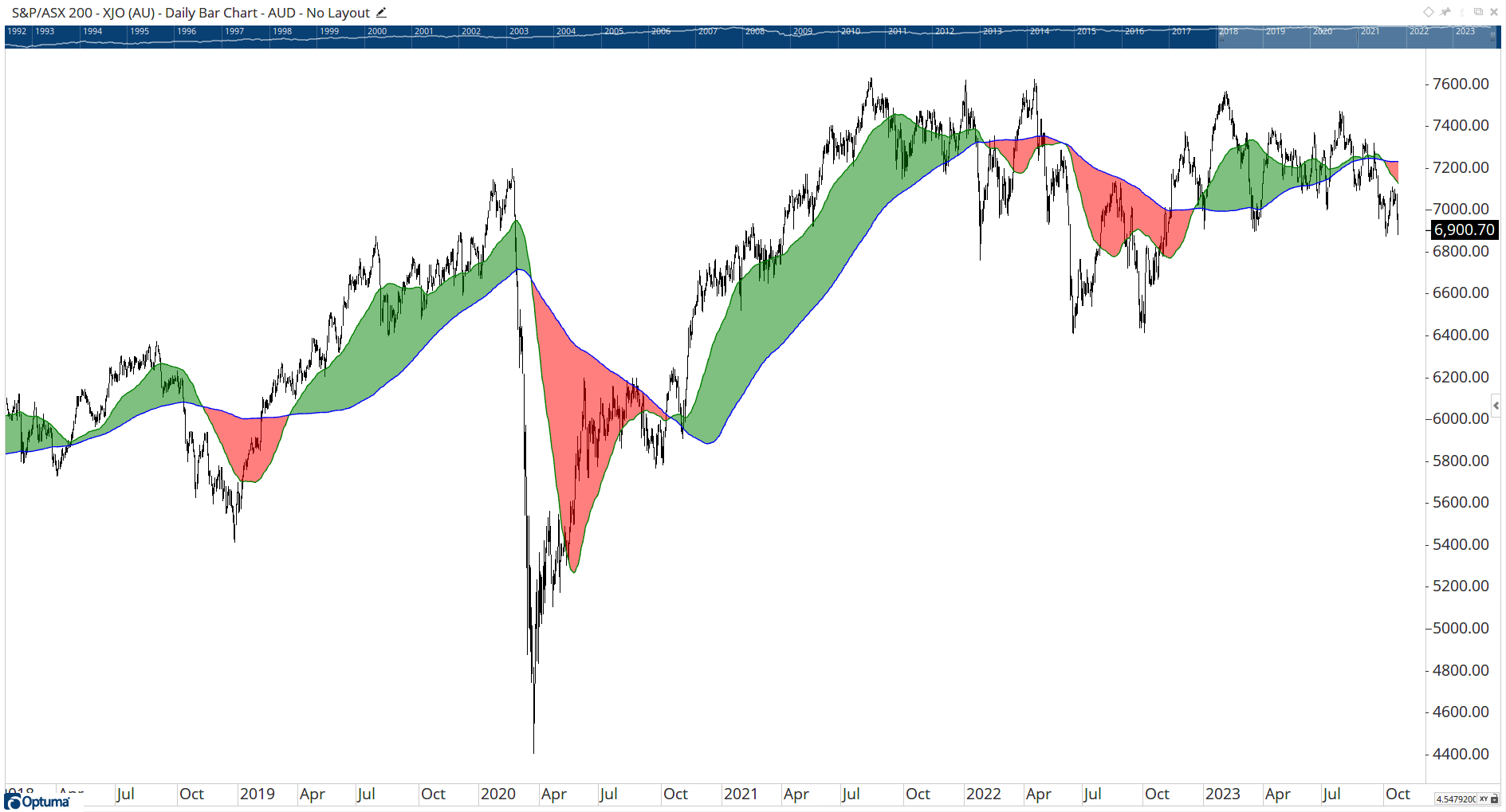Author: Optuma Team Last updated: Oct 23, 2023 05:22

## Overview

The supported properties for this function are:

• Down Colour: Set the colour of the shaded zone when Plot 1 is lower than Plot 2.
• Up Colour: Set the colour of the shaded zone when Plot 1 is higher than Plot 2.
• Offset: Move the shaded zone forward or backwards (measured in bars).
• Transparency: Allows you to adjust the Transparency of the shaded zone.

### Example Scripts

``````Plot1 = MA(BARS=50, STYLE=Simple, CALC=Close);
Plot2 = MA(BARS=200, STYLE=Simple, CALC=Close);
Plot3 = SHADE(Plot1, Plot2, DOWNCOLOUR=Red, UPCOLOUR=Green);
``````

The above script can be used with a Show Plot indicator to produce the following result, with the difference shaded green when the 50MA is above the 200MA, and red when below:To shade zones of the RSI:

``````//Get RSI;
V1=RSI(BARS=14);
Plot1= V1;
Plot1.Colour = Black;
Plot1.LineWidth = 2;
//Set RSI Levels;
Plot2 = 0;
Plot3 = 35;
Plot3.Transparency = 0;
Plot4 = 50;
Plot4.Transparency = 0;
Plot5 = 65;
Plot5.Transparency = 0;
Plot6 = 100;
//Set Colours;